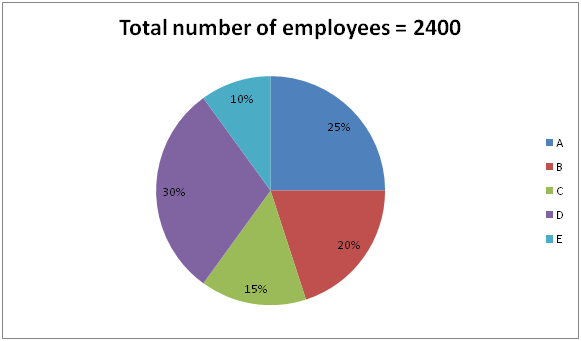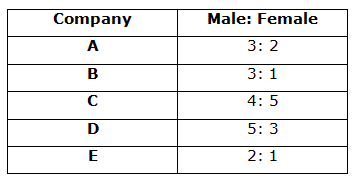# SBI Clerk Pre Quantitative Aptitude (Day-41)

Dear Aspirants, Our IBPS Guide team is providing new series of Quantitative Aptitude Questions for SBI Clerk Prelims 2020 so the aspirants can practice it on a daily basis. These questions are framed by our skilled experts after understanding your needs thoroughly. Aspirants can practice these new series questions daily to familiarize with the exact exam pattern and make your preparation effective.

Start Quiz

Ensure Your Ability Before the Exam – Take SBI Clerk 2020 Prelims Free Mock Test

Data Interpretation

Directions (1 – 5): Study the following information carefully and answer the questions given below.

The pie chart shows the number of employees in 5 different companies.The given table shows the number of male to female employees in five different companies.1) The number of female employees from C is approximately what percent of the number of male employees from D?

a) 44.44%

b) 55.55%

c) 33.33%

d) 22.22%

e) 66.66%

2) What is the average number of male employees in all the companies together?

a) 275

b) 286

c) 294

d) 298

e) None of these

3) What is the ratio of the number of female employees from A to total number of employees from E?

a) 2: 1

b) 1: 1

c) 2: 3

d) 3: 2

e) None of these

4) The ratio of the number of IT to HR employees from B is 7: 5 and the ratio of the number of male IT to HR employees from B is 3: 1. What is the ratio of the number of female employees from IT to HR from B?

a) 1: 11

b) 2: 13

c) 1: 5

d) 2: 9

e) None of these

5) Total number of male employees from E is approximately what percent of the total number of employees from D?

a) 33.33%

b) 22.22%

c) 11.11%

d) 44.44%

e) None of these

Application Sums

6) A invests Rs.4800 at simple interest scheme at x % per annum for 4 years and after 4 years he received the total amount is Rs.7680. If B invests same amount at simple interest scheme at (x + 3)% per annum for 5 years, find the interest amount received by B?

a) Rs.2340

b) Rs.5430

c) Rs.4320

d) Rs.2880

e) None of these

7) A started the business with the investment of Rs.4500 and after 4 months B joined with the business. At the end of the year the total profit is Rs.17000 and A’s profit share is Rs.9000, what is B’s investment?

a) Rs.4000

b) Rs.5000

c) Rs.6000

d) Rs.8000

e) None of these

8) Ratio of the ages of Shon and Sania before 6 years is 4: 5 and the ratio of the age of Shon to Sania after 12 years will be 7: 8. What is the ratio of the present age of Shon to Sania?

a) 5: 6

b) 6: 7

c) 7: 8

d) 4: 5

e) None of these

9) If the perimeter of the square is 112 cm and the surface area of the sphere is 5544 cm2, find the ratio of the side of the square to radius of the sphere?

a) 3: 2

b) 4: 3

c) 2: 1

d) 7: 1

e) None of these

10) A is 150% more efficiency than B and B is 20% less efficiency than C. If C complete one-fourth of the work in 5 days, then in how many days A, B and C together can complete the work?

a) 5(5/19) days

b) 8(5/19) days

c) 4(5/19) days

d) 6(5/19) days

e) None of these

Directions (1 – 5):Required percentage = (200/450) * 100 = 44.44%

Average = (360 + 360 + 160 + 450 + 160) / 5 = 298

Required ratio = 240: 240 = 1: 1

Number of IT employees = 480 * 7/12 = 280

Number of HR employees = 480 * 5/12 = 200

Number of male employees from IT = 360 * ¾ = 270

Number of male employees from HR = 360 * 1/4 =90

Number of female employees from IT = 280 – 270 = 10

Number of female employees from HR = 200 – 90 = 110

Required ratio = 10: 110

= 1: 11

Required percentage = (160/720) * 100 = 22.22%

Directions (6-10) :

SI = P * N * R/100

7680 – 4800 = 4800 * x * 4/100

x = 15%

SI received by B = 4800 * (15 + 3) * 5/100

= 4320

Profit ratio of A and B = 4500 * 12: x * 8

=6750: x

6750/x + 6750 = 9000/17000

9x + 6750 * 9 = 17 * 6750

9x = 54000

x = 6000

(4x + 6 + 12)/(5x + 6 + 12) = 7/8

(4x + 18) * 8 = (5x + 18) * 7

32x + 144 = 35x + 126

3x = 18

x = 6 years

Preset age ratio of Shon to Sania= (4 * 6 + 6):(5 * 6 + 6)

= 30: 36

=5: 6

Perimeter of the square = 112 cm

Side of the square = 112/4 = 28 cm

Surface area of sphere= 4 * 22/7 * r * r

5544 = 4 * 22/7 * r * r

Radius of the sphere = 21 cm

Required ratio = 28: 21

= 4: 3

C complete the whole work in = 4/1 * 5 = 20 days

B/80 = C/100

B/C = 4/5

B = 5/4 * 20 = 25 days

A = 100/250 * 25 = 10 days

A + B + C = 1/20 + 1/25 + 1/10

= 5 + 4 + 10/100

=100/19 days

= 5(5/19) days

 Check Here to View SBI Clerk Prelims 2020 Quantitative Aptitude Questions Day 40 Day 39 Day 38 Click Here for SBI Clerk 2020 – Detailed Exam Notification